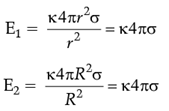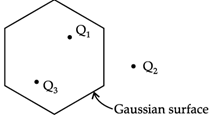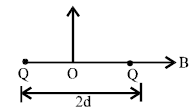# Assertion & Reason Type Questions: Electric Charges & Fields Notes | Study Physics Class 12 - NEET

## NEET: Assertion & Reason Type Questions: Electric Charges & Fields Notes | Study Physics Class 12 - NEET

The document Assertion & Reason Type Questions: Electric Charges & Fields Notes | Study Physics Class 12 - NEET is a part of the NEET Course Physics Class 12.
All you need of NEET at this link: NEET

Directions: In the following questions, A statement of Assertion (A) is followed by a statement of Reason (R). Mark the correct choice as.
Question 1:
Assertion (A): In a non-uniform electric field, a dipole will have translatory as well as rotatory motion.
Reason (R): In a non-uniform electric field, a dipole experiences a force as well as torque.
(a) Both A and R are true and R is the correct explanation of A
(b) Both A and R are true but R is NOT the correct explanation of A
(c) A is true but R is false
(d) A is false and R is true

When an electric dipole is placed in a uniform electric field at an angle q with the field, the dipole experiences a torque. The torque produced by two parallel forces qE acting as couple = τ
τ = qE (2l sin θ)
In case of non-uniform field, force acting on both the ends of the dipole will not be equal. So, there will be a combination of couple and a net force. In this way, dipole will have both rotational as well as linear motion.
So, both assertion and reason are true. Reason also explains the assertion.

Question 2:
Assertion (A): Electric lines of force cross each other.
Reason (R): The resultant electric field at a point is the superimposition of the electric fields at that point.
(a) Both A and R are true and R is the correct explanation of A
(b) Both A and R are true but R is NOT the correct explanation of A
(c) A is true but R is false
(d) A is false and R is true

Electric lines of force never cross each other. If they cross each other, then at that point, we get two directions of electric field at that point, which is not possible. So, the assertion is false. The resultant electric field at a point is a vector sum of the electric fields at that point.

Question 3:
Assertion (A): If two spherical conductors of different radii have the same surface charge densities, then their electric field intensities will be equal.
Reason (R): Surface charge density = Total charge/area
(a) Both A and R are true and R is the correct explanation of A
(b) Both A and R are true but R is NOT the correct explanation of A
(c) A is true but R is false
(d) A is false and R is true

If σ be the surface charge density of the two spheres of radius r and R, then electric fields for the two spheres are respectively:So electric field intensities are equal. The assertion is true.
Surface charged density is charge per unit area = Total charge/area.
So reason is also true.
But the reason does not explain the assertion.

Question 4:
Assertion (A): Three point charges Q1, Q2 and Q3 are shown in the figure. The flux over the Gaussian surface depends on only one charge point.Reason (R): Electric flux depends on the all charges nearby.
(a) Both A and R are true and R is the correct explanation of A
(b) Both A and R are true but R is NOT the correct explanation of A
(c) A is true but R is false
(d) A is false and R is true

According to Gauss’s law, electric flux depends on the enclosed charges only. Here the enclosed charges are Q1 and Q3 only. Hence the assertion is false and the reason is true.

Directions: These questions consist of two statements, each printed as Assertion and Reason. While answering these questions, you are required to choose any one of the following four responses.
Question 5:
Assertion: Electron move away from a region of lower potential to a region of higher potential.
Reason: An electron has a negative charge.
(a) If both Assertion and Reason are correct and the Reason is a correct explanation of the Assertion.
(b) If both Assertion and Reason are correct but Reason is not a correct explanation of the Assertion.
(c) If the Assertion is correct but Reason is incorrect.
(d) If both the Assertion and Reason are incorrect.

Direction of electric field is from region of high potential to low potential & electron or any -ve charged particle will move against the field or lower potential to higher potential.

Question 6:
Assertion: Electric lines of force never cross each other.
Reason: Electric field at a point superimpose to give one resultant electric field.
(a) If both Assertion and Reason are correct and the Reason is a correct explanation of the Assertion.
(b) If both Assertion and Reason are correct but Reason is not a correct explanation of the Assertion.
(c) If the Assertion is correct but Reason is incorrect.
(d) If both the Assertion and Reason are incorrect.

Electric lines of force never cross each other. Electric field at a point add up vectorally to give one resultant electric field. So, they do not have independent existence at the point of superposition so, electric lines of force do not cross each other(crossing of electric lines of force at a point means at a point two fields are having independent existence).

Question 7:
Assertion: In a cavity within a conductor, the electric field is zero.
Reason: Charges in a conductor reside only at its surface.

(a) If both Assertion and Reason are correct and the Reason is a correct explanation of the Assertion.
(b) If both Assertion and Reason are correct but Reason is not a correct explanation of the Assertion.
(c) If the Assertion is correct but Reason is incorrect.
(d) If both the Assertion and Reason are incorrect.

Net field inside the conductor is zero because. by 'virtue of induced charges, applied and induced electric fields are equal and opposite thus the net charge resides on surface only. If any cavity is there inside the conductor, electric field will be zero in it.

Question 8:
Assertion: The tyres of aircraft are slightly conducting.
Reason: If a conductor is connected to ground, the extra charge induced on conductor will flow to ground.

(a) If both Assertion and Reason are correct and the Reason is a correct explanation of the Assertion.
(b)
If both Assertion and Reason are correct but Reason is not a correct explanation of the Assertion.
(c)
If the Assertion is correct but Reason is incorrect.
(d)
If both the Assertion and Reason are incorrect.

Both the statements are independently correct.

Question 9:
Assertion: Coulomb force and gravitational force follow the same inverse-square law.
Reason: Both laws are same in all aspects.
(a) If both Assertion and Reason are correct and the Reason is a correct explanation of the Assertion.
(b)
If both Assertion and Reason are correct but Reason is not a correct explanation of the Assertion.
(c)
If the Assertion is correct but Reason is incorrect.
(d)
If both the Assertion and Reason are incorrect.

Coulomb force and gravitational force follow the same inverse-square law. But gravitational force has only one sign which is always attractive, while coulomb force can be of both signs which are attractive and repulsive.

Question 10:
Assertion: If there exists coulomb attraction between two bodies, both of them may not be charged.
Reason: In coulomb attraction two bodies are oppositely charged.
(a) If both Assertion and Reason are correct and the Reason is a correct explanation of the Assertion.
(b) If both Assertion and Reason are correct but Reason is not a correct explanation of the Assertion.
(c) If the Assertion is correct but Reason is incorrect.
(d) If both the Assertion and Reason are incorrect.

Coulomb attraction exists even when one body is charged, and the other is uncharged.

Question 11:
Assertion: A metallic shield in form of a hollow shell may be built to block an electric field.
Reason: In a hollow spherical shield, the electric field inside it is zero at every point
(a) If both Assertion and Reason are correct and the Reason is a correct explanation of the Assertion.
(b) If both Assertion and Reason are correct but Reason is not a correct explanation of the Assertion.
(c) If the Assertion is correct but Reason is incorrect.
(d) If both the Assertion and Reason are incorrect.

The electrostatic shielding is possible by metallic conductor.

Question 12:
Assertion: Consider two identical charges placed distance 2d apart, along x-axis.The equilibrium of a positive test charge placed at the point O midway between them is stable for displacements along the x-axis.
Reason: Force on test charge is zero.
(a) If both Assertion and Reason are correct and the Reason is a correct explanation of the Assertion.
(b) If both Assertion and Reason are correct but Reason is not a correct explanation of the Assertion.
(c) If the Assertion is correct but Reason is incorrect.
(d) If both the Assertion and Reason are incorrect.

If +ve charge is displaced along x-axis, then net force will always act in a direction opposite to that of displacement and the test charge will always come back to its original position.

Question 13:
Assertion: A uniformly charged disc has a pin hole at its centre. The electric field at the centre of the disc is zero.
Reason: Disc can be supposed to be made up of many rings. Also electric field at the centre of uniformly charged ring is zero.
(a) If both Assertion and Reason are correct and the Reason is a correct explanation of the Assertion.
(b) If both Assertion and Reason are correct but Reason is not a correct explanation of the Assertion.
(c) If the Assertion is correct but Reason is incorrect.
(d) If both the Assertion and Reason are incorrect.

The electric field due to disc is superposition of electric field due to its constituent ring as given in Reason.

Question 14:
Assertion: Electric lines of field cross each other.
Reason: Electric field at a point superimpose to give one resultant electric field.
(a) If both Assertion and Reason are correct and the Reason is a correct explanation of the Assertion.
(b) If both Assertion and Reason are correct but Reason is not a correct explanation of the Assertion.
(c) If the Assertion is correct but Reason is incorrect.
(d) If both the Assertion and Reason are incorrect.

Two field lines never intersect.

Question 15:
Assertion: On going away from a point charge or a small electric dipole, electric field decreases at the same rate in both the cases.
Reason: Electric field is inversely proportional to square of distance from the charge or an electric dipole.
(a) If both Assertion and Reason are correct and the Reason is a correct explanation of the Assertion.
(b)
If both Assertion and Reason are correct but Reason is not a correct explanation of the Assertion.
(c)
If the Assertion is correct but Reason is incorrect.
(d)
If both the Assertion and Reason are incorrect.

For electric dipole, the field falls as cube of distance from the dipole center. But for point charge, it falls as square of distance. So, assertion and reason are both incorrect.

The document Assertion & Reason Type Questions: Electric Charges & Fields Notes | Study Physics Class 12 - NEET is a part of the NEET Course Physics Class 12.
All you need of NEET at this link: NEETUse Code STAYHOME200 and get INR 200 additional OFF

## Physics Class 12

156 videos|419 docs|213 tests

### How to Prepare for NEET

Read our guide to prepare for NEET which is created by Toppers & the best Teachers

Track your progress, build streaks, highlight & save important lessons and more!

,

,

,

,

,

,

,

,

,

,

,

,

,

,

,

,

,

,

,

,

,

;